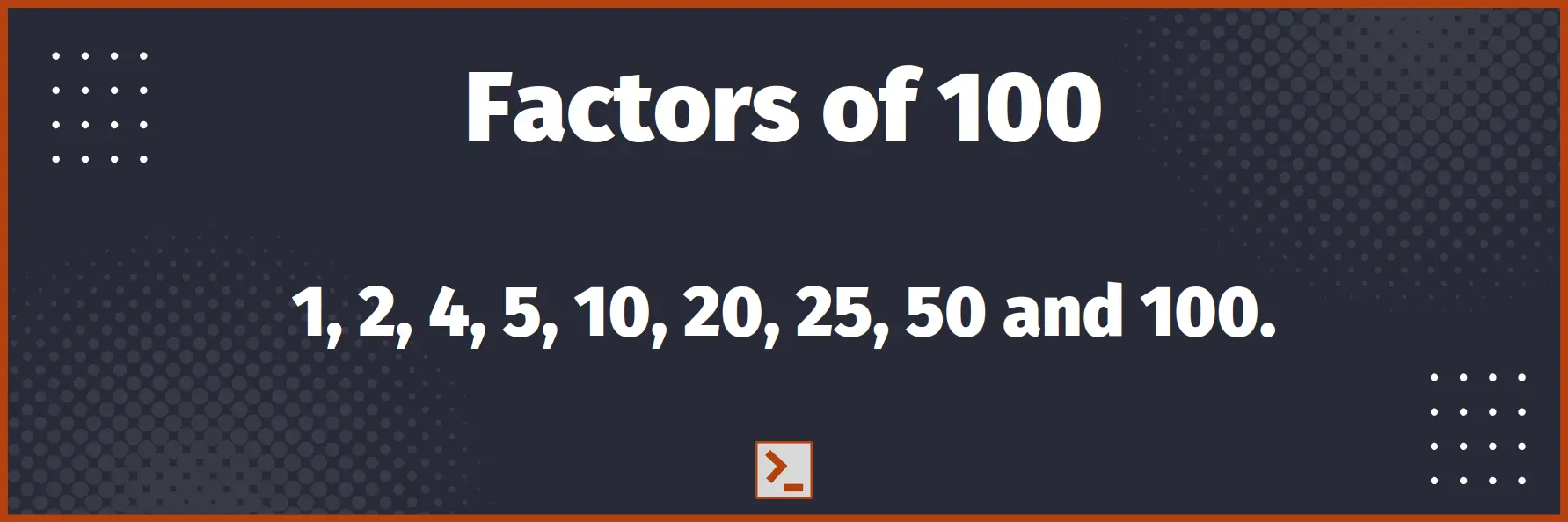# Factors of a Natural Number

And a program in Rust to list all the factors of a natural number

## What are Factors of a number

Factors or divisors of a natural number, say n, is a natural number, say m, that perfectly divides the number n. That is, n % m = 0. It can also be written that n = km, where k is also a natural number.

For Example : 1, 2, and 4 are factors of 4, but 3 is not a factor of 4## Naive Approach

Naive or brute force approach is to traverse all the numbers from 1 to n, and add the number to vector if it divides the given number. Function for this approach in Rust is :

```fn list_factors(number:i128) -> Vec<i128>{    // Initialize factors Vector    let mut factors : Vec<i128> = Vec::new();
// Check all the numbers from 1 to n, both inclusive    for i in 1..(number+1) {        if number % i == 0 {            // Push the number to factors, if it divides number            factors.push(i);        }    }
// Return the factors Vector as answer    return factors;}```

Program with driver code

```use std::io;
fn list_factors(number:i128) -> Vec<i128>{    // Initialize factors Vector    let mut factors : Vec<i128> = Vec::new();
// Check all the numbers from 1 to n, both inclusive    for i in 1..(number+1) {        if number % i == 0 {            // Push the number to factors, if it divides number            factors.push(i);        }    }
// Return the factors Vector as answer    return factors;}
// Driver Code
fn main() {    // Read and parse number to i128    let mut input = String::new();    io::stdin().read_line(&mut input).unwrap();    let number : i128 = input.trim().parse().unwrap();
println!("{:?}", list_factors(number));}```

Input

100

Output

[1, 2, 4, 5, 10, 20, 25, 50, 100]

Time Complexity : O(n)
Space Complexity : O( sqrt(n) )

Note : We can print the number instead of storing into the array or vector, to reduce Space Complexity to O(1) but it is rarely useful. Vector of factors is far more useful in real applications, so I will demonstrate Vector, instead of printing directly.

## Efficient Approach

If you look the condition for factor carefully, that is

n = k.m

So, both k and m are factors of n, and not only m. So, all the factors of number are in pair

For example, factors of 100 are ( 1, 100 ), ( 2, 50 ), ( 4, 25 ), ( 5, 20 ) and ( 10, 10 ). Clearly,If m is a factor of n, n/m is also a factor of n

Using this property, we only have to check for numbers till square root of n, because it can't be possible to get n, as a product of 2 numbers greater than square root of n.

Function for this approach :

```fn list_factors(number:i128) -> Vec<i128>{    // Initialize factors Vector    let mut factors : Vec<i128> = Vec::new();
let mut i : i128 = 1;
// Check till i is less than or equal to square root n    while i*i <= number{        if number % i == 0 {            factors.push(i);
// to prevent duplication, if number is perfect square            if i*i != number {                factors.push(number / i);            }        }        i+=1;    }
// It is generally useful to sort the vector    // And it will not affect our time complexity,    // Because logarithmic time is much less than square root time complexity
factors.sort();
// Return the factors Vector as answer    return factors;}```

Use the same driver code.

Input

100

Output

[1, 2, 4, 5, 10, 20, 25, 50, 100]

Time Complexity : O( sqrt(n) )
Space Complexity : O( sqrt(n) )

Note : Repeating, We can print the number instead of storing into the array or vector, to reduce Space Complexity to O(1) but it is rarely useful. Vector of factors is far more useful in real applications, so I will demonstrate Vector, instead of printing directly.

## Conclusion

Factors or divisors of a natural number are the numbers that perfectly divides it. In this article we made a program to list all the factors of a natural number in Rust, and also optimized it to square root time complexity. Here is the optimized function for easy access

`fn list_factors(number:i128) -> Vec<i128>{    let mut factors : Vec<i128> = Vec::new();    let mut i : i128 = 1;    while i*i <= number{        if number % i == 0 {            factors.push(i);            if i*i != number { factors.push(number / i); }        }        i+=1;    }    factors.sort();    return factors;}`

Thank You